• 2021-05-25 06:01:45

#define _CRT_SECURE_NO_WARNINGS

#include

#include

#include

#define MAX_SIZE 255    //定义字符串的最大长度

typedef unsigned char SString[MAX_SIZE];//数组第一个保存长度

//BF

int BFMatch(char *s,char *p)

{

int i,j;

i=0;

while(i < strlen(s))

{

j=0;

while(s[i]==p[j]&&j < strlen(p))

{

i++;

j++;

}

if(j==strlen(p))

return i-strlen(p);

i=i-j+1;                //指针i回溯

}

return -1;

}

//getNetx

void getNext(char *p,int *next)

{

int j,k;

next=-1;

j=0;

k=-1;

while(j < strlen(p)-1)

{

if(k==-1||p[j]==p[k])    //匹配的情况下,p[j]==p[k]

{

j++;

k++;

next[j]=k;

}

else

{                  //p[j]!=p[k]

k=next[k];

}

}

}

//KMP

int KMPMatch(char *s,char *p)

{

int next;

int i,j;

i=0;

j=0;

getNext(p,next);

while(i < strlen(s))

{

if(j==-1||s[i]==p[j])

{

i++;

j++;

}

else

{

j=next[j];       //消除了指针i的回溯

}

if(j==strlen(p))

{

return i-strlen(p);

}

}

return -1;

}

int main()

{

int a, b;

char s[MAX_SIZE], p[MAX_SIZE];

printf("请输入模式串：");

scanf("%s", &s);

printf("请输入子串：");

scanf("%s", &p);

a = BFMatch(s, p);

b = KMPMatch(s, p);

if(a != -1)

{

printf("使用BF算法：%d\n", a);

}

else

{

printf("未匹配\n");

}

if(b != -1)

{

printf("使用KMP算法：%d\n", a);

}

else

{

printf("未匹配\n");

}

system("pause");

}

更多相关内容
• #include #include #include #include //以下为KMP算法void get_next(char * T, int next[]) //修正前的next数组{int i = 1, j = 0;next = -1;next = 0;int m = strlen(T);while (i{if (j == -1 || T[j] == T...

#include

#include

#include

#include

//以下为KMP算法

void get_next(char * T, int next[]) //修正前的next数组

{

int i = 1, j = 0;

next = -1;

next = 0;

int m = strlen(T);

while (i

{

if (j == -1 || T[j] == T[i])

{

++i;

++j;

next[i] = j;

}

else j = next[j];

}

}

void get_nextval(char * T, int nextval[]) //修正后的nextval数组

{

int i = 1, j = 0;

nextval = -1;

nextval = 0;

int m = strlen(T);

while (i

{

if (j == -1 || T[j] == T[i])

{

++i;

++j;

if (T[i] != T[j]) nextval[i] = j;

else nextval[i] = nextval[j];

}

else j = nextval[j];

}

}

int Index_KMP(char * S, char * T, int pos, int next[]) //逐项比较

{

int j = 0, i = pos, lens = strlen(S), lent = strlen(T);

get_next(T, next);

while (i < lens && j < lent)

{

if (S[i] == T[j] || j == -1)

{

i++; j++;

}

else j = next[j];

}

if (j >= lent) return i - lent;

else return -1;

}

//以下为BF算法

int Pos_BF(char * S, char * T)

{

int pos = 1;

for (int i = 0; i < strlen(S); i++)

{

if (S[i] == T)

return pos;

else

pos++;

}

return 0;

}

int Index_BF(char * S, char * T)

{

int i = 0, j = 0;

int lens = strlen(S), lent = strlen(T);

while (i <= lens -1 && j <= lent -1 )

{

if (S[i] == T[j])

{

++i;

++j;

}

else

{

i = i - j + 1;

j = 0;

}

}

//跳出循环有两种可能，i=lens说明已经遍历完主串，匹配失败；j=lent,说明子串遍历完成，在主串中成功匹配

if (j == lent) {

return i - lent + 1;

}

//运行到此，为i==lens的情况

return -1;

}

int main()

{

char S;

char T;

printf("请输入两个字符串进行匹配：\n");

printf("请输入母串：");

scanf("%s", S);

printf("请输入匹配串：");

scanf("%s", T);

//此处开始测试KMP算法

printf("\n**********************KMP算法**************************\n");

int m;

int *next = (int *)malloc((strlen(T) + 1)*sizeof(int)); //修正前的next数组

int *nextval = (int *)malloc((strlen(T) + 1)*sizeof(int)); //修正后的nextval数组

get_next(T, next);

printf("修正前next数组为：");

for (m = 0; m

{

printf("%d ", next[m] + 1);

}

get_nextval(T, nextval);

printf("\n修正后的nextval数组为：");

for (m = 0; m

{

printf("%d ", nextval[m] + 1);

}

int t1 = Index_KMP(S, T, 0, nextval);

if (t1 == -1) printf("\n无匹配项！\n");

else

{

printf("\n在第%d项开始匹配成功\n", t1 + 1);

}

//此处开始测试BF算法

printf("\n**********************BF算法**************************\n");

int t2 = Index_BF(S, T);

printf("\n在第%d项开始匹配成功\n", t2);

system("pause");

return 0;

}原文：https://www.cnblogs.com/zhengyu-ahu/p/12038827.html

展开全文• 这次我们介绍串的BF模式匹配和KMP模式匹配

子串（模式串）的定位操作通常称作串的模式匹配

其中包含最初始的BF算法（Brute-Force）即简单匹配算法或者称作朴素的模式匹配算法，利用穷举法的思路

另一种就是改进后的KMP算法，还有对KMP算法的一种优化算法

现在先展示第一种BF算法的代码及解决思路：

#define _CRT_SECURE_NO_WARNINGS 1
#define MAXSIZE 255
#define ERROR 0
#include<stdio.h>
#include<stdlib.h>

typedef struct {
char ch[MAXSIZE];
int length;
}SString;

int main()
{
int compare(SString S, SString T, int pos);
SString	S;
SString T;//定义主串和模式串（子串）,分别为S,T
int i = 1;//i表示主串第一个元素的数组下标
//比较串通常从数组下标为1开始，0位置不存储或者通常存放数组的长度
//这里为了方便数组S.ch和T.ch不存放任何数据，长度事先定好并存在S.length和T.length中
int j = 1;//j表示子串第一个元素的数组下标
printf("请输入主串长度\n");
scanf("%d%*c", &S.length);//使用%*c吃掉回车，不然scanf读取回车存在缓存区
//下一次使用scanf输入char类型的元素会先读取缓存区的换行符（\n），影响多次scanf使用
//这里有好几种解决方法，具体请参考《解决scanf读取回车问题》
printf("请输入主串元素\n");
while (i < S.length+1)//这里数组长度的定义是你输入的总元素的个数，不是所谓的总长度
//最开始我们的0下标位置没有存放，所以i从1开始，循环S.length次，所以<S.length+1
//这种比较不确定时候掰手指头带几个数字算算~~~~
{
scanf("%c", &S.ch[i]);//输入前面已经定好个数的主串元素，记住输入时候不要加空格！！！！！
i++;
}
printf("请输入子串长度\n");//这里就不用考虑回车的问题了，%c情况下才会读取，%d无影响
scanf("%d%*c", &T.length);//使用%*c吃掉回车，不然scanf读取回车存在缓存区，影响多次scanf使用
printf("请输入子串元素\n");
while (j < T.length + 1)
{
scanf("%c", &T.ch[j]);
j++;
}
int pos = 1;//pos是比较起点，也就是从主串哪一位置开始和子串比较
int s = 0;//s保存比较函数的返回值
printf("请输入比较起始点：\n");
scanf("%d", &pos);
if (pos<1 || pos>S.length)//记住比较最小从1开始，最大不能超过主串总长
//因为我们把元素从1开始存放，所以从哪个元素开始位序和下标一样，不用担心不符合问题
{
exit(ERROR);
}
else
{
s = compare(S, T, pos);
}
if (s != 0)
printf("字串从第%d位开始匹配成功",s);
else
printf("未匹配成功");

return 0;
}

int compare(SString S, SString T, int pos)//BF算法，i每次要回溯
{
int i = pos;//i代表起时从哪个位置开始比较
int j = 1;//j代子串的元素位置
while (i <= S.length&& j <= T.length)//如果i，j分别小于等于各自长度就可以继续比，大于就没得比了~
{
if (S.ch[i] == T.ch[j])//如果相等
{
++i;
++j;//i和j都++，都准备开始比较各自的下一个元素
}
else
{
i = i - j + 2;//i回溯到当前开始比较位置的下一位置
//这里理解为（i-j+1）+1，前面括号里面是刚开始比较的i的位置序号，后面加一表示往后移动一位
//从后面一位开始，其位置元素作为新的比较起点开始比较
j = 1;//j回溯到起始位置,每次匹配失败后子串就从头开始比较
}
}
if (j > T.length) //最后如果比较成功了，子串对应的j达到了最后一位，即T.length
//但是要记住，你最后一个元素匹配成功进入的if语句还有一个++i和++j，所以最后j=T.length+1
return (i - T.length);//上面说明了，i最后还有个++，最后i值为比完的最后一位的下一位对应的i
else
return 0;
}

每次比较后，i都要回溯，j也得从第一位开始重新比较，效率其实非常低，于是一种新的算法腾空出现，就是最初的KMP算法。

他的核心思想就是i不回溯，一遍到底，j回溯到合适位置，不是每次从头开始

KMP的主函数和BF的差不多，先展示其匹配函数：

int KMP_cmp(SString S, SString T, int pos)//KMP算法
{
int i = pos;
int j = 1;
int next[MAXSIZE];//定义next数组
nextval(T, next);//为next数组赋值
while (i <= S.length && j <= T.length)
{
if (j==0||S.ch[i] == T.ch[j])//多增加了j==0的判断
{
++i;
++j;
}
else
{
j = next[j];//i不回溯，j回溯到适当位置
}
}
if (j > T.length)
return (i - T.length);
else
return 0;
}

再就是创建不完美的next数组的函数：

​
void nextval(SString T, int* next)//最原始的KMP算法的next数组
//如果碰到aaaaab这种子串，会被赋值012345，增加了不必要的回溯
{
int i, k;
i = 1;
k = 0;
next = 0;
while (i < T.length)
{
if (k == 0 || T.ch[i] == T.ch[k])
{
++i;
++k;
next[i] = k;

}
else
k = next[k];
}
}

​

先给大家简单分析一下k和i的含义和关系（改进后的照样继续分析即可）：

首先是next数组生成时候我们把if和else抽出来分析：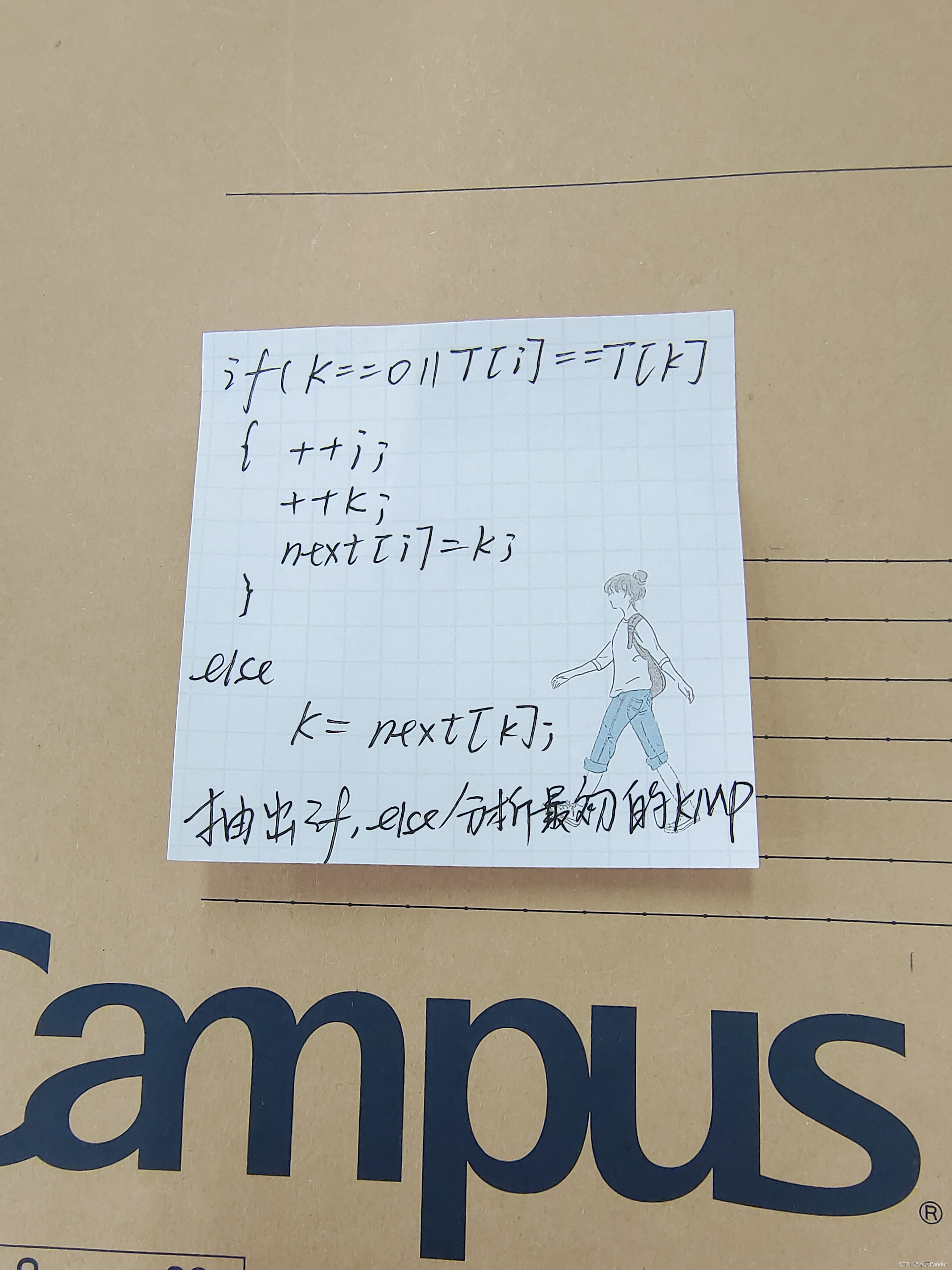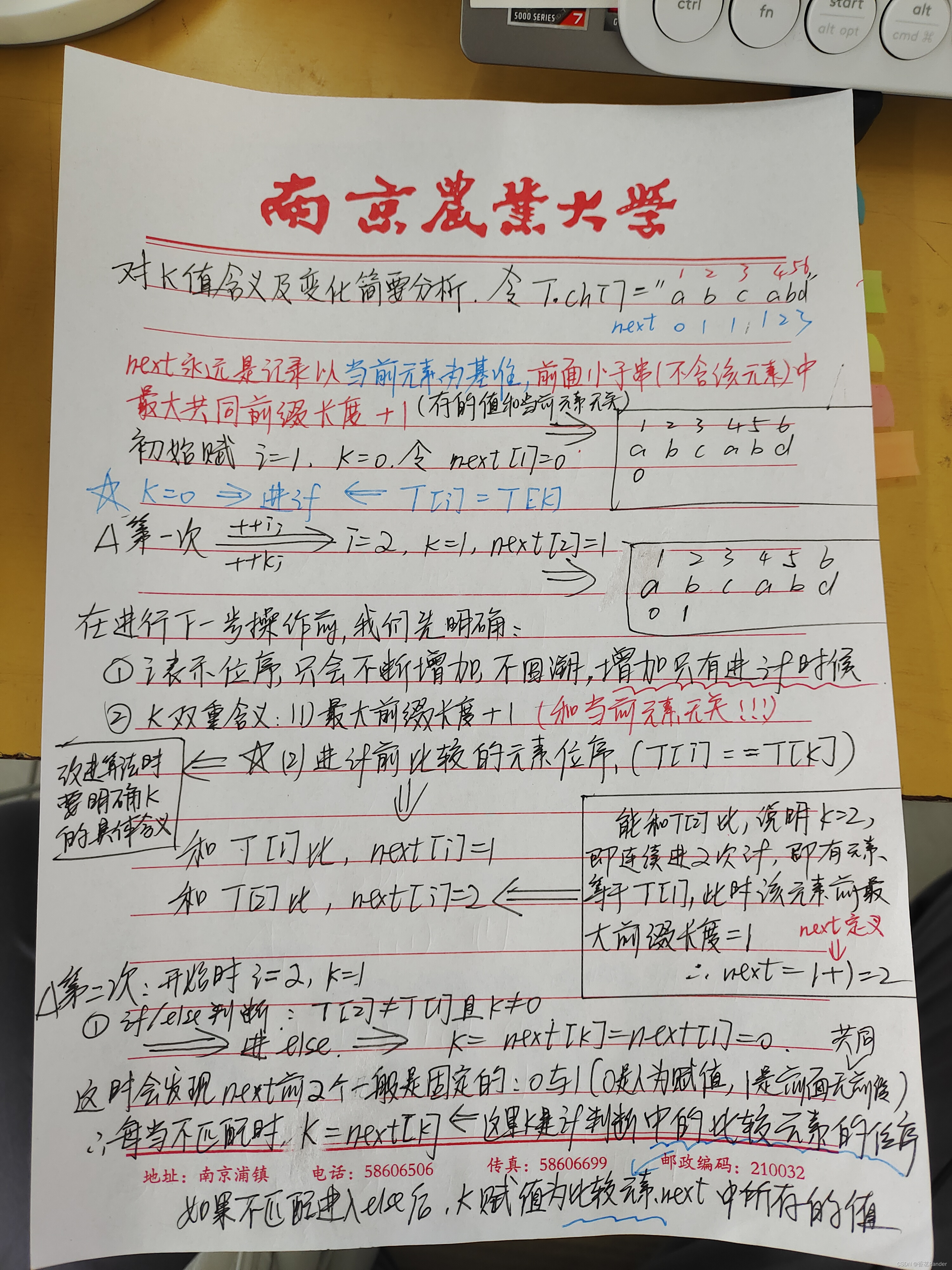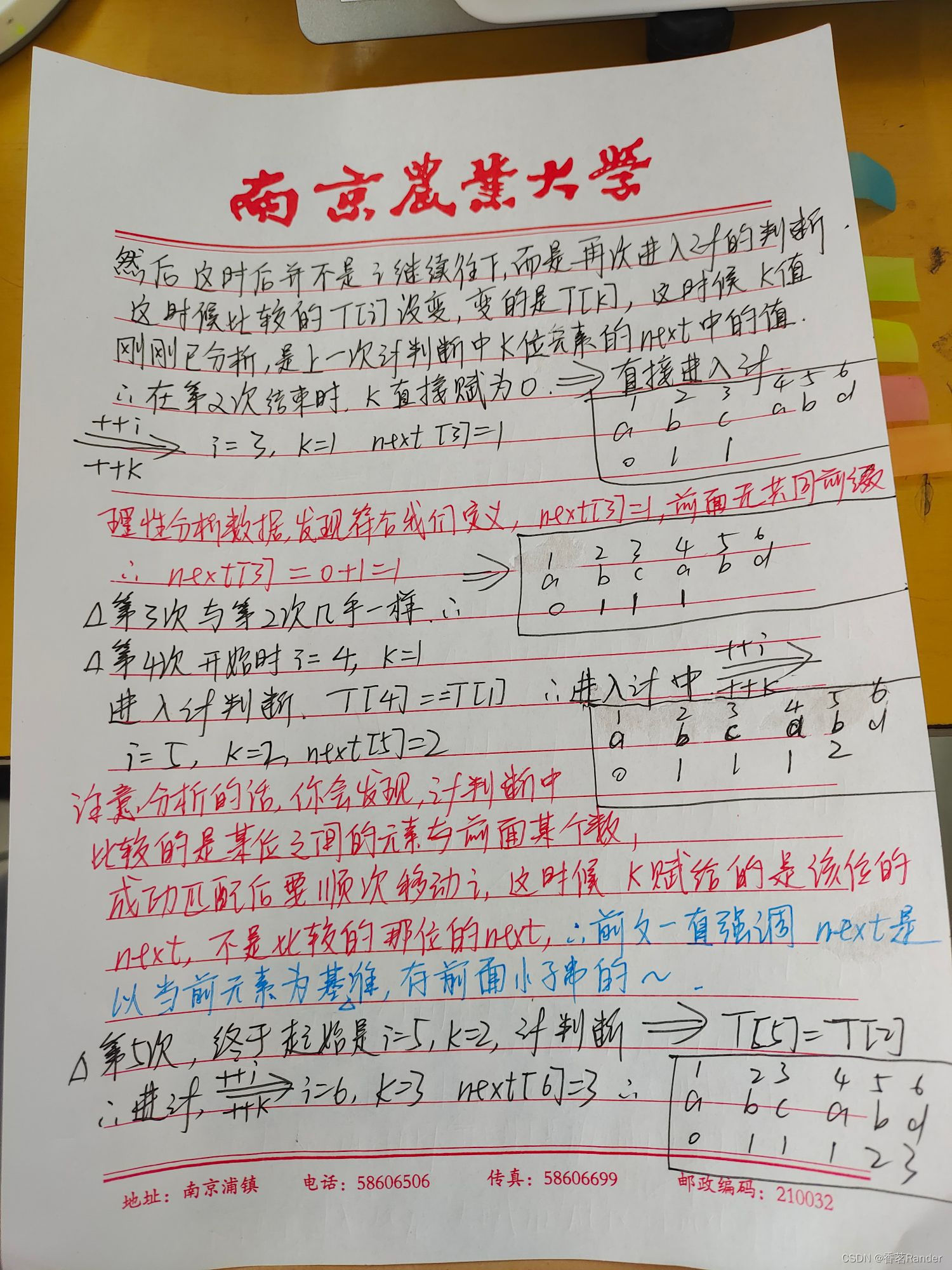现在把最完美的KMP算法展示给大家：

（解决了前面重复元素的多次k回溯问题）

#define _CRT_SECURE_NO_WARNINGS 1
#define MAXSIZE 255
#define ERROR 0
#include<stdio.h>
#include<stdlib.h>

typedef struct {
char ch[MAXSIZE];
int length;
}SString;

int main()
{

int KMP_cmp(SString S, SString T, int pos);
SString	S;
SString T;//定义主串和模式串（子串）
int i = 1;
int j = 1;

printf("请输入主串长度\n");
scanf("%d%*c", &S.length);//使用%*c吃掉回车，不然scanf读取回车存在缓存区，影响多次scanf使用
printf("请输入主串元素\n");
while (i < S.length + 1)
{
scanf("%c", &S.ch[i]);
i++;
}
printf("请输入子串长度\n");//这里就不用考虑回车的问题了，%c情况下才会读取，%d无影响
scanf("%d%*c", &T.length);//使用%*c吃掉回车，不然scanf读取回车存在缓存区，影响多次scanf使用
printf("请输入子串元素\n");
while (j < T.length + 1)
{
scanf("%c", &T.ch[j]);
j++;
}
int pos = 1;
int s = 0;
printf("请输入比较起始点：\n");
scanf("%d", &pos);
if (pos<1 || pos>S.length)//记住比较最小从1开始，最大不能超过主串总长
//因为我们把元素从1开始存放，所以从哪个元素开始位序和下标一样，不用担心不符合问题
{
exit(ERROR);
}
else
{
s = KMP_cmp(S, T, pos);
}
if (s != 0)
printf("字串从第%d位开始匹配成功", s);
else
printf("未匹配成功");

return 0;
}

//主函数和BF算法一致，关键在于一个next数组的构建，他存放着j要回溯到的位置

void nextval(SString T, int *next)
{
int i, k;
i = 1;//i表示元素的位序
k = 0;//k代表当前i位置元素的最大公共前缀+1
//例如abcabd，next值为011123
next = 0;//第一个元素的next值人为先定义为0
while (i < T.length)
{
if (k == 0 || T.ch[i] == T.ch[k])//如果k==0或者i位置和k位置的元素值相等
{
++i;
++k;
if (T.ch[i] != T.ch[k])//如果i位置的值和k位置的值不等，也就是外面if中以k==0条件进来的
next[i] = k;//把此时k的值赋给i位置元素的next
else
next[i] = next[k];//如果相等i位置的next和k位置的next相等
}
else
k = next[k];//如果既不相等k又不等于0，k就被赋值为k位置的next
}
}

int KMP_cmp(SString S, SString T, int pos)//KMP算法
{
int i = pos;
int j = 1;
int next[MAXSIZE];//定义next数组
nextval(T, next);//为next数组赋值
while (i <= S.length && j <= T.length)
{
if (j==0||S.ch[i] == T.ch[j])//多增加了j==0的判断
{
++i;
++j;
}
else
{
j = next[j];//i不回溯，j回溯到适当位置
}
}
if (j > T.length)
return (i - T.length);
else
return 0;
}

下面是输入输出结果：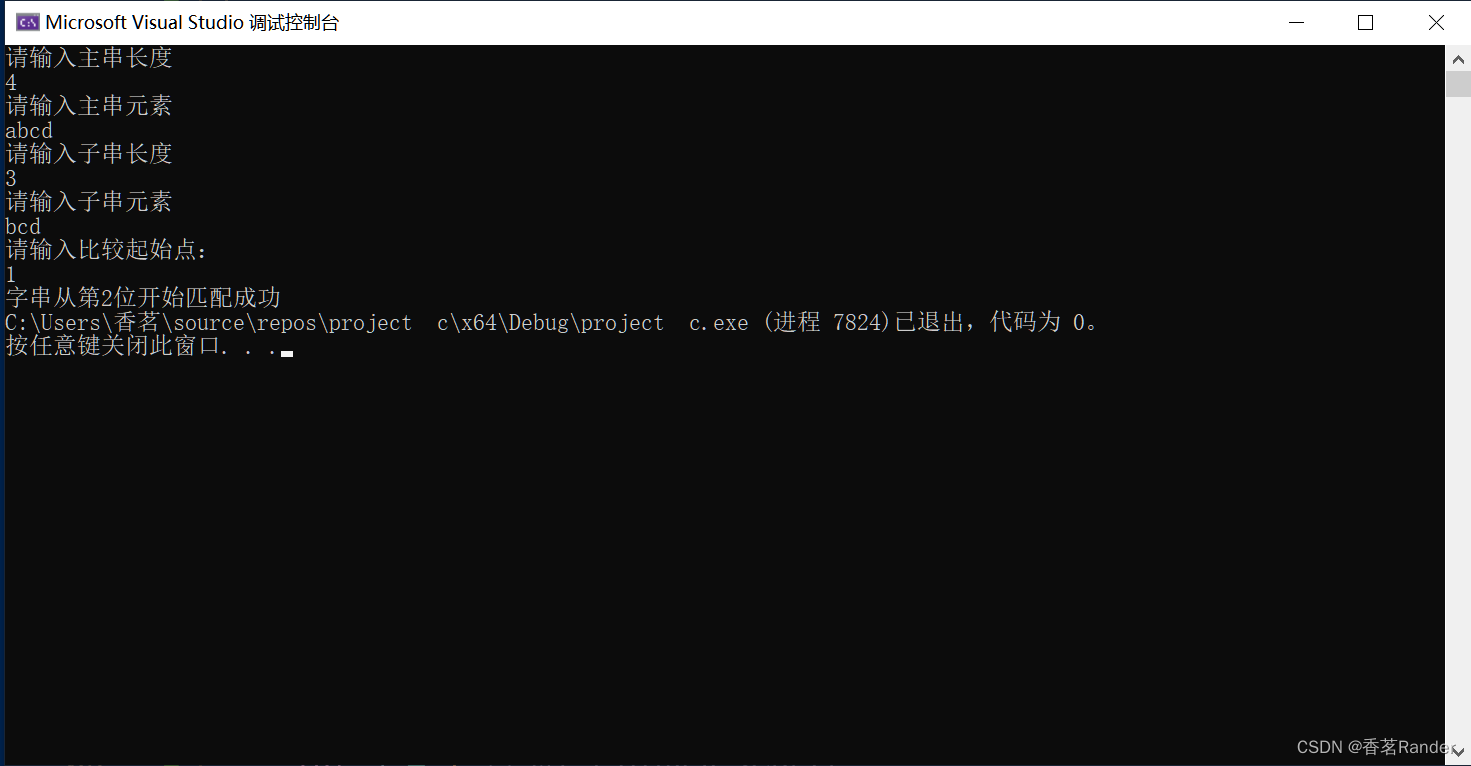下面展示用数组第一位（下标为0）存储数组长度的相同算法（BF与KMP）：

#define MAXSIZE 255
#define ERROR 0
#include<stdio.h>
#include<stdlib.h>

typedef struct {
char ch[MAXSIZE];
}SString;

int main()
{
//int compare(SString S, SString T, int pos);
int KMP_cmp(SString S, SString T, int pos);
SString	S;
SString T;
int i = 1;
int j = 1;
char x, y;//先把串元素的值赋给x，y再把其赋给数组对应下标位置，这样能方便终止输入
printf("请输入主串元素(输入'#'停止，每输入一个元素用enter换行)\n");
scanf("%c%*c", &x);
for (i = 1; x != '#'; i++)
{
S.ch[i] = x;
scanf("%c%*c", &x);
}
S.ch = i - 1;//数组下标为0的位置存放数组的长度
fflush(stdin);//清空缓存区的所有字符，这里因为最后输入#后面接了一个换行，不清除后面子串第一个接收的就是换行符
printf("请输入子串元素(输入'#'停止，每输入一个元素用enter换行)\n");
scanf("%c%*c", &y);
for (j = 1; y != '#'; j++)
{
T.ch[j] = y;
scanf("%c%*c", &y);
}
T.ch = j - 1;
int pos = 1;//pos是比较起点，也就是从主串哪一位置开始和子串比较
int s = 0;//s保存比较函数的返回值
printf("请输入比较起始点：\n");
scanf("%d", &pos);
if (pos<1 || pos>S.ch)//记住比较最小从1开始，最大不能超过主串总长
//因为我们把元素从1开始存放，所以从哪个元素开始位序和下标一样，不用担心不符合问题
{
exit(ERROR);
}
else
{
//s = compare(S, T, pos);
s = KMP_cmp(S, T, pos);
}
if (s != 0)
printf("字串从第%d位开始匹配成功", s);
else
printf("未匹配成功");

return 0;
}

//int compare(SString S, SString T, int pos)//BF算法，i每次要回溯
//{
//	int i = pos;//i代表起时从哪个位置开始比较
//	int j = 1;//j代子串的元素位置
//	while (i <= S.ch && j <= T.ch)//如果i，j分别小于等于各自长度就可以继续比，大于就没得比了~
//	{
//		if (S.ch[i] == T.ch[j])//如果相等
//		{
//			++i;
//			++j;//i和j都++，都准备开始比较各自的下一个元素
//		}
//		else
//		{
//			i = i - j + 2;//i回溯到当前开始比较位置的下一位置
//			//这里理解为（i-j+1）+1，前面括号里面是刚开始比较的i的位置序号，后面加一表示往后移动一位
//			//从后面一位开始，其位置元素作为新的比较起点开始比较
//			j = 1;//j回溯到起始位置,每次匹配失败后子串就从头开始比较
//		}
//	}
//	if (j > T.ch) //最后如果比较成功了，子串对应的j达到了最后一位，即T.length
//		//但是要记住，你最后一个元素匹配成功进入的if语句还有一个++i和++j，所以最后j=T.length+1
//		return (i - T.ch);//上面说明了，i最后还有个++，最后i值为比完的最后一位的下一位对应的i
//	else
//		return 0;
//}
//
void nextval(SString T, int *next)
{
int i, k;
i = 1;//i表示元素的位序
k = 0;//k代表当前i位置元素的最大公共前缀+1
//例如abcabd，next值为011123
next = 0;//第一个元素的next值人为先定义为0
while (i < T.ch)
{
if (k == 0 || T.ch[i] == T.ch[k])//如果k==0或者i位置和k位置的元素值相等
{
++i;
++k;
if (T.ch[i] != T.ch[k])//如果i位置的值和k位置的值不等，也就是外面if中以k==0条件进来的
next[i] = k;//把此时k的值赋给i位置元素的next
else
next[i] = next[k];//如果相等i位置的next和k位置的next相等
}
else
k = next[k];//如果既不相等k又不等于0，k就被赋值为k位置的next
}
}

int KMP_cmp(SString S, SString T, int pos)//KMP算法
{
int i = pos;
int j = 1;
int next[MAXSIZE];//定义next数组
nextval(T, next);//为next数组赋值
while (i <= S.ch && j <= T.ch)
{
if (j==0||S.ch[i] == T.ch[j])//多增加了j==0的判断
{
++i;
++j;
}
else
{
j = next[j];//i不回溯，j回溯到适当位置
}
}
if (j > T.ch)
return (i - T.ch);
else
return 0;
}

下面是输入输出结果：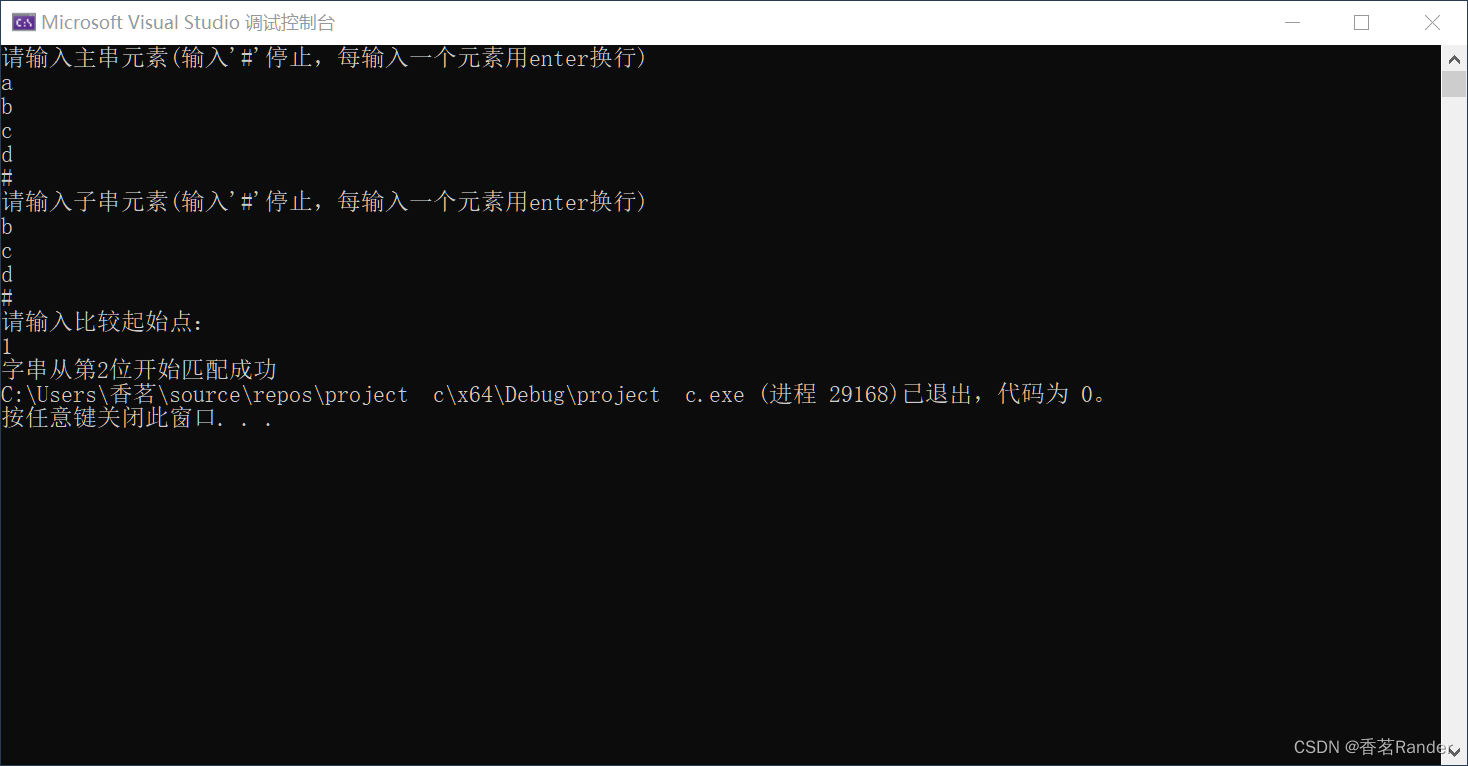时间复杂度：(m,n分别是主串和子串的长度）

BF算法： O(m*n)

KMP算法 O(m+n)

KMP的思想和next数组创建建议多独立分析，先搞清思想再来用代码实现~~

独立研究next数组的创建过程------为什么需要、为什么这么建立

展开全文数据结构
• BF算法和KMP算法是字符串的两种主要的模式匹配算法 1.BF算法，即暴力(Brute Force)算法，是普通的模式匹配算法，BF算法的思想就是将目标串S的第一个字符与模式串T的第一个字符进行匹配，若相等，则继续比较S的第二...

BF算法和KMP算法是字符串的两种主要的模式匹配算法

1.BF算法，即暴力(Brute Force)算法，是普通的模式匹配算法，BF算法的思想就是将目标串S的第一个字符与模式串T的第一个字符进行匹配，若相等，则继续比较S的第二个字符和 T的第二个字符；若不相等，则比较S的第二个字符和T的第一个字符，依次比较下去，直到得出最后的匹配结果。

如主串和子串的下标为i和j。如果两位相等即a[i]==b[j]， i++，j++，都后移一位。但是如果两位不相等，子串j会回到初始位置，而主串会回到上一次开始匹配的下一位，即i=i-j+1，再次匹配。

下列为BF算法：

//BF算法
int BF(String S,String T){
int i=1;// 主串的位置
int j=1;// 模式串的位置
int sum1=0;
while(j<=T.length &&i<=S.length){
if(T.ch[j-1]==S.ch[i-1]){// 当两个字符相同，就比较下一个
++i;
++j;
sum1++;
}
else{
i=i-j+2;// 一旦不匹配，i后退
j=1;// j归1
sum1++;//比较次数
}
}
printf("BF比较%d\n",sum1);
if(j>T.length)return i-T.length;
else return -1;

}

next计算：

给大家示例：例如： 模式串 a b a a b c a c             next值 0 1 1 2 2 3 1 2

next数组的求解方法是：第一位的next值为0，第二位的next值为1，后面求解每一位的next值时，根据前一位进行比较。 首先将前一位与其next值对应的内容进行比较， 如果相等，则该位的next值就是前一位的next值加上1； 如果不等，向前继续寻找next值对应的内容来与前一位进行比较，直到找到某个位上内容的next值对应的内容与前一位相等为止，则这个位对应的值加上1即为需求的next值； 如果找到第一位都没有找到与前一位相等的内容，那么需求的位上的next值即为1。

//得到next值
void getnext(String T,int next[]){
// 求子串T的next函数值并存入数组next
int i=1;
int j=0;
next=0;
while(i<T.length){
if(j==0||T.ch[i]==T.ch[j]){
++i;++j;
next[i]=j;
}
else{
j=next[j];
}
}
}

2.KMP算法：即是对BF算法的一种优化，所实现的功能是一样的。其优点是利用已经部分匹配这个有效信息，保持i指针不回溯，通过修改j指针，让模式串尽量地移动到有效的位置j指针返回到对应的next值。

//KMP算法
int  KMP(String S,String T,int next[]){
int i=1;// 主串的位置
int j=1;// 模式串的位置
int sum2=0;
while(j<=T.length&&i<=S.length){
if(j==0||T.ch[j-1]==S.ch[i-1]){
++i;
++j;// 继续比较后继字符
sum2++;
}
else {
j=next[j];j回到指定位置
sum2++;//比较次数
}
}
printf("KMP比较%d\n",sum2);
if(j>T.length)return i-T.length;  // 匹配成功
else return -1;

}

完整的代码：

#include <stdio.h>
#include<string.h>
#define Maxsize 100
typedef struct Lnode{
char ch[Maxsize];
int length;
}String;
//得到next值
void getnext(String T,int next[]){
int i=1;
int j=0;
next=0;
while(i<T.length){
if(j==0||T.ch[i]==T.ch[j]){
++i;++j;
next[i]=j;
}
else{
j=next[j];
}
}
}
//BF算法
int BF(String S,String T){
int i=1;
int j=1;
int sum1=0;
while(j<=T.length &&i<=S.length){
if(T.ch[j-1]==S.ch[i-1]){
++i;
++j;
sum1++;
}
else{
i=i-j+2;
j=1;
sum1++;
}
}
printf("BF比较%d\n",sum1);
if(j>T.length)return i-T.length;
else return -1;

}
//KMP算法
int  KMP(String S,String T,int next[]){
int i=1;
int j=1;
int sum2=0;
while(j<=T.length&&i<=S.length){
if(j==0||T.ch[j-1]==S.ch[i-1]){
++i;
++j;
sum2++;
}
else {
j=next[j];
sum2++;
}
}
printf("KMP比较%d\n",sum2);
if(j>T.length)return i-T.length;
else return -1;

}
//主函数
int main(){
String T;
String S;
scanf("%s",&S.ch);
scanf("%s",&T.ch);
S.length=strlen(S.ch);
T.length=strlen(T.ch);
int next[Maxsize];
getnext( T,next);
int t=BF(S, T);
int m= KMP( S, T, next);
printf("BF在第%d位置\n",t);
printf("KMP在第%d位置\n",m);
return 0;
} 

展开全文• KMP算法的关键是利用匹配失败后的信息，尽量减少模式串与主串的匹配次数以达到快速匹配的目的。具体实现就是实现一个next()函数，函数本身包含了模式串的局部匹配信息。时间复杂度O(m+n)。 简单理解就是：KMP算法是...数据结构
• 一.BF算法 1.原理 暴力查找 逐个匹配主串字符，然后模式串j值回溯到1重新匹配 2.代码实现 二.KMP算法 1.原理 核心是避免不必要的回溯 问题是由模式串决定，不是目标串决定 只需要将j值模式串中j的位置回溯到next[j]...数据结构
• 基于BF和KMP的串模式匹配算法设计与实现(C语言).rar
•数据结构
• ## KMP算法详细解析（c语言篇）

千次阅读 多人点赞 2022-03-22 13:00:12
KMP算法是一种改进的字符串匹配算法，由D.E.Knuth，J.H.MorrisV.R.Pratt提出的，因此人们称它为克努特—莫里斯—普拉特操作（简称KMP算法）。KMP算法的核心是利用匹配失败后的信息，尽量减少模式串与主串的匹配...leetcode 开发语言 后端
• KMP算法在模式与主串之间存在许多“部分匹配”的情况下，比BF算法快。(注意，接下来的串都是以下标为1作为起始储存位置。)下面说一下实现代码：首先是预定义和类型定义：#define MAXLEN 100typedef struct{char ch...
•c++
• 基于BF和KMP的串模式匹配算法设计与实现(C语言)
• BF算法   BF算法也称朴素算法，思想简单，但效率较低。代码如下： #include<stdio.h> #include<string.h> //s是主串，p是子串，从s串的pos位置开始搜索 int BF(char* s,char* p,int pos)//时间复杂度O...
• 字符串匹配 【问题描述】 对于字符串ST，若T是S子串，返回T在S中的位置（T的首字符在S中...采用直接穷举法求解，称为BF算法。该算法从S的每一个字符开始查找，看T是否会出现。例如，S=“aababcde”,T=“abcd”: ...数据结构 字符串
• ## 最详BF算法和KMP算法

多人点赞 热门讨论 2022-06-03 15:44:02
本篇文章主要时写出了BF算法和KMP算法，以及总结了两种算法的区别与优缺点。开发语言 数据结构
• 以前看过kmp算法，当时接触后总感觉好深奥啊，抱着数据结构的数啃了一中午，最终才大致看懂，后来提起kmp也只剩下“奥，它是做模式匹配的”这点干货。最近有空，翻出来算法导论看看，原来就是这么简单(下不说程序...
• printf("KMP算法的时间%f",duration); cout使用KMP算法："; if(p1==-1) cout主串中没有匹配的子串"; else cout从第"个字符开始找起,第一次出现子串的位置为"; start1=clock(); while(j){ p2=BF...
• BF算法实现 #include<stdio.h> int Index_BF(int *a,int *b){ int i,j; i=j=0; while(i<10&&j<4){ if(a[i]==b[j]){ i++; j++; }else{ i=(i-j)+2; j=1; } } return i-4;...
• KMP算法是一种改进的字符串匹配算法，由D.E.Knuth，J.H.MorrisV.R.Pratt提出的，因此人们称它为克努特—莫里斯—普拉特操作（简称KMP算法）。KMP算法的核心是利用匹配失败后的信息，尽量减少模式串与主串的匹配...c++ 开发语言
• BF和KMP匹配算法一、BF匹配算法二、KMP匹配算法 一、BF匹配算法 BF模式匹配算法，又称朴素模式匹配算法，简单模式匹配算法，暴力匹配算法。对于字符串，现有一个主串一个子串，那么子串在主串中的定位操作通常称为...算法 数据结构 模式匹配算法 字符串
• BF算法（简单匹配法，穷举思路） int match(SString S,SString T){ int i=1,j=1; while(i<=S.length&&j<=T.length){ if(s.ch[i]==t.ch[i]){i++;j++}//主串字串以此匹配下一个字符 else {i=i-j...数据结构
• 最近，需要复习KMP算法的next数组，然后回头看半年多后的我回头看半年多前自己综合别人内容写的介绍。 没错，自己也看不懂。然后，自己再根据自己的理解写了一下理解透彻的笔记，方便理解记忆，当然，以前的代码解释...数据结构
•开发语言
• 一、BF算法BF算法是普通的模式匹配算法，其基本思想就是将目标串的第一个字符与模式串的第一个字符进行匹配。若相等，则继续比较第二个字符；若不相等，则比较目标串的第二个字符模式串的第一个字符。依次比较下去......